28 篇文章 1 订阅

# 找工作知识储备(3)---从头说12种排序算法：原理、图解、动画视频演示、代码以及笔试面试题目中的应用

9366人阅读  评论(10)  收藏  举报

1. 前言
2. 一插入排序
1. 1算法简介
2. 2算法描述和分析
3. 3算法图解flash演示视频演示
4. 4算法代码
5.  5考察点重点和频度分析
6. 6笔试面试例题
3. 二二分插入排序
1. 1算法简介
2. 2算法描述和分析
3. 3算法图解flash演示视频演示
4. 4算法代码
5.  5考察点重点和频度分析
6. 6笔试面试例题
4. 三希尔排序
1. 1算法简介
2. 2算法描述
3. 3算法图解flash演示视频演示
4. 4算法代码
5. 5考察点重点和频度分析
6. 6笔试面试例题
5. 四选择排序
6. 五冒泡排序
1. 1算法简介
2. 2算法描述
3. 3算法图解flash演示视频演示
4. 4算法代码
5. 5考察点重点和频度分析
6. 6笔试面试例题
1. 例题1
2. 例题2
7. 六鸡尾酒排序双向冒泡排序
8. 七快速排序
1. 1算法简介
2. 2算法描述和分析
3. 3算法图解flash演示视频演示
4. 4算法代码
5. 5考察点重点和频度分析
6. 6笔试面试例题
9. 八堆排序
1. 1算法简介
2. 2算法描述
3. 3算法图解flash演示视频演示
4. 4算法代码
5. 5考察点重点和频度分析
6. 6笔试面试题
10. 九归并排序
1. 1算法简介
2. 2算法描述
3. 3算法图解flash演示视频演示
4. 4算法代码
5. 5考察点重点和频度分析
6. 6笔试面试题
11. 十桶排序
1. 1算法简介
2. 2算法描述和分析
3. 3算法图解flash演示视频演示
4. 4算法代码
5. 5考察点重点和频度分析
6. 6笔试面试题
12. 十一计数排序
1. 1算法简介
2. 2算法描述和分析
3. 3算法图解flash演示视频演示
4. 4算法代码
5. 5考察点重点和频度分析
6. 6笔试面试题
1. 例题1
13. 十二基数排序
14. 总结

# 0、前言

从这一部分开始直接切入我们计算机互联网笔试面试中的重头戏算法了，初始的想法是找一条主线，比如数据结构或者解题思路方法，将博主见过做过整理过的算法题逐个分析一遍(博主当年自己学算法就是用这种比较笨的刷题学的，囧)，不过又想了想，算法这东西，博主自己学的过程中一直深感，基础还是非常重要的，很多难题是基础类数据结构和题目的思想综合发散而来。比如说作为最基本的排序算法就种类很多，而事实上笔试面试过程中发现掌握的程度很一般，有很多题目，包括很多算法难题，其母题或者基本思想就是基于这些经典算法的，比如说快排的partition算法，比如说归并排序中的思想，比如说桶排序中桶的思想。
这里对笔试面试最常涉及到的12种排序算法(包括插入排序、二分插入排序、希尔排序、选择排序、冒泡排序、鸡尾酒排序、快速排序、堆排序、归并排序、桶排序、计数排序和基数排序)进行了详解。每一种算法都有基本介绍、算法原理分析、图解/flash演示/视频演示、算法代码、笔试面试重点分析、笔试面试题等板块，希望能帮助大家真正理解这些排序算法，并能使用这些算法的思想解决一些题。不多说了，下面就进入正题了。

# 一、插入排序

## 1）算法简介

插入排序（Insertion Sort）的算法描述是一种简单直观的排序算法。它的工作原理是通过构建有序序列，对于未排序数据，在已排序序列中从后向前扫描，找到相应位置并插入。插入排序在实现上，通常采用in-place排序（即只需用到O(1)的额外空间的排序），因而在从后向前扫描过程中，需要反复把已排序元素逐步向后挪位，为最新元素提供插入空间。

## 2）算法描述和分析

一般来说，插入排序都采用in-place在数组上实现。具体算法描述如下：

1、从第一个元素开始，该元素可以认为已经被排序

2、取出下一个元素，在已经排序的元素序列中从后向前扫描

3、如果该元素（已排序）大于新元素，将该元素移到下一位置

4、重复步骤3，直到找到已排序的元素小于或者等于新元素的位置

5、将新元素插入到该位置后

6、重复步骤2~5

如果目标是把n个元素的序列升序排列，那么采用插入排序存在最好情况和最坏情况。最好情况就是，序列已经是升序排列了，在这种情况下，需要进行的比较操作需(n-1)次即可。最坏情况就是，序列是降序排列，那么此时需要进行的比较共有n(n-1)/2次。插入排序的赋值操作是比较操作的次数减去(n-1)次。平均来说插入排序算法复杂度为O(n^2)。因而，插入排序不适合对于数据量比较大的排序应用。但是，如果需要排序的数据量很小，例如，量级小于千，那么插入排序还是一个不错的选择。 插入排序在工业级库中也有着广泛的应用，在STL的sort算法和stdlib的qsort算法中，都将插入排序作为快速排序的补充，用于少量元素的排序（通常为8个或以下）。

## 3）算法图解、flash演示、视频演示

### 图解：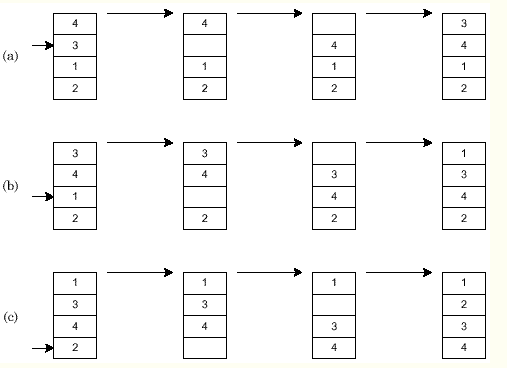## 4）算法代码

[cpp]  view plain copy
1. void insertion_sort(int array[], int first, int last)
2.  {
3.         int i,j;
4.         int temp;
5.         for (i = first+1; i<=last;i++)
6.         {
7.                 temp = array[i];
8.                 j=i-1;
9.
10.                 //与已排序的数逐一比较，大于temp时，该数后移
11.                 while((j>=first) && (array[j] > temp))  //当first=0，j循环到-1时，由于[[短路求值]]，不会运算array[-1]
12.                 {
13.                         array[j+1] = array[j];
14.                         j--;
15.                 }
16.                 array[j+1] = temp;      //被排序数放到正确的位置
17.
18.         }
19.  }

## 5）考察点，重点和频度分析

把插入排序放在第一个的原因是因为其出现的频度不高，尤其是这里提到的直接排序算法，基本在笔试的选择填空问时间空间复杂度的时候才可能出现。毕竟排序速度比较慢，因此算法大题中考察的次数比较比较少。

## 6）笔试面试例题

### 例题1、

[cpp]  view plain copy
1. template<typename T>
2. struct list_node
3. {
4.     struct list_node<T> *next;
5.     T value;
6. };
7. template<typename T>
8. struct _list
9. {
11.     int size;
12. };
13. template<typename T>
24.     }
25. }

### 例题2、

A.快速排序     B.冒泡排序   C.直接插入排序   D.堆排序

# 二、二分插入排序

## 1）算法简介

二分（折半）插入（Binary insert sort)排序是一种在直接插入排序算法上进行小改动的排序算法。其与直接排序算法最大的区别在于查找插入位置时使用的是二分查找的方式，在速度上有一定提升。

## 2）算法描述和分析

一般来说，插入排序都采用in-place在数组上实现。具体算法描述如下：

1、从第一个元素开始，该元素可以认为已经被排序

2、取出下一个元素，在已经排序的元素序列中二分查找到第一个比它大的数的位置

3、将新元素插入到该位置后

4、重复上述两步

1）稳定

2）空间代价：O(1)

3）时间代价：插入每个记录需要O(log i)比较，最多移动i+1次，最少2次。最佳情况O(n log n)，最差和平均情况O(n^2)

二分插入排序是一种稳定的排序。当n较大时，总排序码比较次数比直接插入排序的最差情况好得多，但比最好情况要差，所元素初始序列已经按排序码接近有序时，直接插入排序比二分插入排序比较次数少。二分插入排序元素移动次数与直接插入排序相同，依赖于元素初始序列。

## 3）算法图解、flash演示、视频演示

### 图解：## 4）算法代码

[cpp]  view plain copy
1. void BinInsertSort(int a[], int n)
2. {
3.         int key, left, right, middle;
4.         for (int i=1; i<n; i++)
5.         {
6.                 key = a[i];
7.                 left = 0;
8.                 right = i-1;
9.                 while (left<=right)
10.                 {
11.                         middle = (left+right)/2;
12.                         if (a[middle]>key)
13.                                 right = middle-1;
14.                         else
15.                                 left = middle+1;
16.                 }
17.
18.                 for(int j=i-1; j>=left; j--)
19.                 {
20.                         a[j+1] = a[j];
21.                 }
22.
23.                 a[left] = key;
24.         }
25. }

## 5）考察点，重点和频度分析

这个排序算法在笔试面试中出现的频度也不高，但毕竟是直接排序算法的一个小改进算法，同时二分查找又是很好的思想，有可能会在面试的时候提到，算法不难，留心一下就会了。

## 6）笔试面试例题

### 例题1、

A、二分插入排序         B、堆排序         C、冒泡排序            D、快速排序

### 例题2、

(1)冒泡排序；(2)选择排序；(3)插入排序；(4)二分插入排序；(5)快速排序；(6)堆排序；(7)归并排序；

# 三、希尔排序

## 2）算法描述

1、先取一个小于n的整数d1作为第一个增量，把文件的全部记录分成d1个组。

2、所有距离为d1的倍数的记录放在同一个组中，在各组内进行直接插入排序。

3、取第二个增量d2<d1重复上述的分组和排序，

4、直至所取的增量dt=1(dt<dt-l<…<d2<d1)，即所有记录放在同一组中进行直接插入排序为止。

希尔排序的时间复杂度与增量序列的选取有关，例如希尔增量时间复杂度为O(n^2)，而Hibbard增量的希尔排序的时间复杂度为O(N^(5/4))，但是现今仍然没有人能找出希尔排序的精确下界。

## 3）算法图解、flash演示、视频演示

### 图解：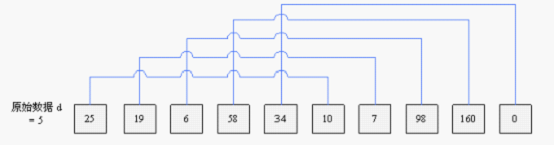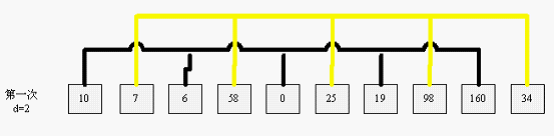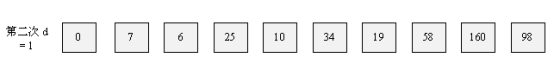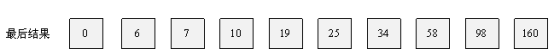## 4）算法代码

[cpp]  view plain copy
1. #include <stdio.h>
2.
3. int main()
4. {
5.      const int n = 5;
6.      int i, j, temp;
7.      int gap = 0;
8.      int a[] = {5, 4, 3, 2, 1};
9.      while (gap<=n)
10.      {
11.           gap = gap * 3 + 1;
12.      }
13.      while (gap > 0)
14.      {
15.          for ( i = gap; i < n; i++ )
16.          {
17.              j = i - gap;
18.              temp = a[i];
19.              while (( j >= 0 ) && ( a[j] > temp ))
20.              {
21.                  a[j + gap] = a[j];
22.                  j = j - gap;
23.              }
24.              a[j + gap] = temp;
25.          }
26.          gap = ( gap - 1 ) / 3;
27.      }
28.  }

## 5）考察点，重点和频度分析

事实上希尔排序算法在笔试面试中出现的频度也不比直接插入排序高，但它的时间复杂度并不是一个定值，所以偶尔会被面试官问到选择的步长和时间复杂度的关系，要稍微有点了解吧。算法大题中使用该方法或者其思想的题也不多。

## 6）笔试面试例题

### 例题1、

程序略，需要O(n^2)次的比较

# 四、选择排序

## 1）算法简介

选择排序(Selection sort)是一种简单直观的排序算法。它的工作原理如下。首先在未排序序列中找到最小（大）元素，存放到排序序列的起始位置，然后，再从剩余未排序元素中继续寻找最小（大）元素，然后放到已排序序列的末尾。以此类推，直到所有元素均排序完毕。

## 2）算法描述和分析

n个记录的文件的直接选择排序可经过n-1趟直接选择排序得到有序结果：

1、初始状态：无序区为R[1..n]，有序区为空。

2、第i趟排序(i=1,2,3...n-1)

3、前n-1趟结束，数组有序化了

选择排序的交换操作介于0(n-1)次之间。选择排序的比较操作为n(n-1)/2次之间。选择排序的赋值操作介于03(n-1)次之间。比较次数O(n^2),比较次数与关键字的初始状态无关，总的比较次数N=(n-1)+(n-2)+...+1=n*(n-1)/2。 交换次数O(n),最好情况是，已经有序，交换0次；最坏情况是，逆序，交换n-1次。 交换次数比冒泡排序少多了，由于交换所需CPU时间比比较所需的CPU时间多，n值较小时，选择排序比冒泡排序快。

 最差时间复杂度 О(n²) 最优时间复杂度 О(n²) 平均时间复杂度 О(n²) 最差空间复杂度 О(n) total, O(1)

## 3）算法图解、flash演示、视频演示

### 图解：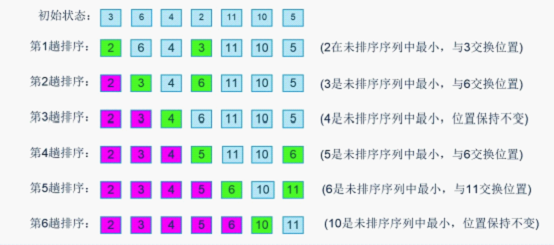## 4）算法代码

[cpp]  view plain copy
1. void selection_sort(int *a, int len)
2. {
3.     register int i, j, min, t;
4.     for(i = 0; i < len - 1; i ++)
5.     {
6.         min = i;
7.         //查找最小值
8.         for(j = i + 1; j < len; j ++)
9.             if(a[min] > a[j])
10.                 min = j;
11.         //交换
12.         if(min != i)
13.         {
14.             t = a[min];
15.             a[min] = a[i];
16.             a[i] = t;
17.         }
18.     }
19. }

## 5）考察点，重点和频度分析

就博主看过的笔试面试题而言，选择算法也大多出现在选择填空中，要熟悉其时间和空间复杂度，最好最坏的情况分别是什么，以及在那种情况下，每一轮的比较次数等。

## 例题2、

下述几种排序方法中，平均查找长度（ASL）最小的是

# 五、冒泡排序

## 1）算法简介

冒泡排序是一种简单的排序算法。它重复地走访过要排序的数列，一次比较两个元素，如果他们的顺序错误就把他们交换过来。走访数列的工作是重复地进行直到没有再需要交换，也就是说该数列已经排序完成。这个算法的名字由来是因为越小的元素会经由交换慢慢“浮”到数列的顶端。

## 2）算法描述

1、比较相邻的元素。如果第一个比第二个大，就交换他们两个。

2、对每一对相邻元素作同样的工作，从开始第一对到结尾的最后一对。在这一点，最后的元素应该会是最大的数。

3、针对所有的元素重复以上的步骤，除了最后一个。

4、持续每次对越来越少的元素重复上面的步骤，直到没有任何一对数字需要比较。

冒泡排序是与插入排序拥有相等的执行时间，但是两种法在需要的交换次数却很大地不同。在最坏的情况，冒泡排序需要O(n^2)次交换，而插入排序只要最多O(n)交换。冒泡排序的实现（类似下面）通常会对已经排序好的数列拙劣地执行（O(n^2)），而插入排序在这个例子只需要O(n)个运算。因此很多现代的算法教科书避免使用冒泡排序，而用插入排序取代之。冒泡排序如果能在内部循环第一次执行时，使用一个旗标来表示有无需要交换的可能，也有可能把最好的复杂度降低到O(n)。在这个情况，在已经排序好的数列就无交换的需要。若在每次走访数列时，把走访顺序和比较大小反过来，也可以稍微地改进效率。有时候称为往返排序，因为算法会从数列的一端到另一端之间穿梭往返。

 最差时间复杂度 O(n^2) 最优时间复杂度 O(n) 平均时间复杂度 O(n^2) 最差空间复杂度 总共O(n)，需要辅助空间O(1)

## 3）算法图解、flash演示、视频演示

### 图解：## 4）算法代码

[cpp]  view plain copy
1. #include <stdio.h>
2. void bubbleSort(int arr[], int count)
3.   {
4.       int i = count, j;
5.       int temp;
6.
7.       while(i > 0)
8.       {
9.          for(j = 0; j < i - 1; j++)
10.          {
11.              if(arr[j] > arr[j + 1])
12.              {   temp = arr[j];
13.                  arr[j] = arr[j + 1];
14.                  arr[j + 1] = temp;
15.              }
16.          }
17.          i--;
18.      }
19.
20.  }
21.
22.  int main()
23.  {
24.      //测试数据
25.      int arr[] = {5, 4, 1, 3, 6};
26.      //冒泡排序
27.      bubbleSort(arr, 5);
28.      //打印排序结果
29.      int i;
30.      for(i = 0; i < 5; i++)
31.          printf("%4d", arr[i]);
32. }

## 5）考察点，重点和频度分析

一般我们学到的第一个排序算法就是冒泡排序，不得不说，这个还真是一个很常见的考点，平均时间空间复杂度，最好最坏情况下的时间空间复杂度，在不同情况下每一趟的比较次数，以及加标志位减少比较次数等，都是需要注意的地方。

## 6）笔试面试例题

### 例题1、

答：冒泡排序的比较和交换次数将最大，都是1+2++n-1=n(n-1)/250×99=4545

### 例题2、

把一个字符串的大写字母放到字符串的后面，各个字符的相对位置不变，不能申请额外的空间。

事实上，这道题放到冒泡排序这里不知道是不是特别合适，只是有一种解法是类似冒泡的思想，如下解法一

#### 解法一、

[cpp]  view plain copy
1. #include <stdio.h>
2. #include <string.h>
3. //题目以及要求：把一个字符串的大写字母放到字符串的后面，
4. //各个字符的相对位置不变，不能申请额外的空间。
5. //判断是不是大写字母
6. int isUpperAlpha(char c){
7. if(c >= 'A' && c <= 'Z'){
8. return 1;
9. }
10. return 0;
11. }
12. //交换两个字母
13. void swap(char *a, char *b){
14. char temp = *a;
15. *a = *b;
16. *b = temp;
17. }
18. char * mySort(char *arr, int len){
19. if(arr == NULL || len <= 0){
20. return NULL;
21. }
22. int i = 0, j = 0, k = 0;
23. for(i = 0; i < len; i++){
24. for(j = len - 1 - i; j >= 0; j--){
25. if(isUpperAlpha(arr[j])){
26. for(k = j; k < len - i - 1; k++){
27. swap(&arr[k], &arr[k + 1]);
28. }
29. break;
30. }
31. //遍历完了字符数组，但是没发现大写字母，所以没必要再遍历下去
32. if(j == 0 && !isUpperAlpha(arr[j])){
33. //结束;
34.                            return arr;
35. }
36. }
37. }
38. //over:
39. return arr;
40. }
41. int main(){
43. printf("%s\n", mySort(arr, strlen(arr)));
44. return 0;
45. }

#### 解法二。

1、两个指针p1p2，从后往前扫描

2p1遇到一个小写字母时停下， p2遇到大写字母时停下，两者所指向的char交换

3p1， p2同时往前一格

[cpp]  view plain copy
1. #include <stdio.h>
2. #include <string.h>
3. //判断是不是大写字母
4. int isUpperAlpha(char c){
5. if(c >= 'A' && c <= 'Z'){
6. return 1;
7. }
8. return 0;
9. }
10. //交换两个字母
11. void swap(char *a, char *b){
12. char temp = *a;
13. *a = *b;
14. *b = temp;
15. }
16. char * Reorder(char *arr, int len){
17. if(arr == NULL || len <= 0){
18. return NULL;
19. }
20. int *p1 = arr;
21. int *p2 = arr;
22. While(p1<arr+len && p2<arr+len){
23. While( isUpperAlpha(*p1) ){
24. P1++;
25. }
26. While( !isUpperAlpha(*p2) ){
27. P2++;
28. }
29. swap(p1, p2)
30. }
31. //结束
32. return arr;
33. }
34. int main(){
36. printf("%s\n", Reorder(arr, strlen(arr)));
37. return 0;
38. }

# 六、鸡尾酒排序/双向冒泡排序

## 1）算法简介

鸡尾酒排序等于是冒泡排序的轻微变形。不同的地方在于从低到高然后从高到低，而冒泡排序则仅从低到高去比较序列里的每个元素。他可以得到比冒泡排序稍微好一点的效能，原因是冒泡排序只从一个方向进行比对(由低到高)，每次循环只移动一个项目。

## 2）算法描述和分析

1、依次比较相邻的两个数，将小数放在前面，大数放在后面；

2、第一趟可得到：将最大数放到最后一位。

3、第二趟可得到：将第二大的数放到倒数第二位。

4、如此下去，重复以上过程，直至最终完成排序。

鸡尾酒排序最糟或是平均所花费的次数都是O(n^2)，但如果序列在一开始已经大部分排序过的话，会接近O(n)

 最差时间复杂度 O(n^2) 最优时间复杂度 O(n) 平均时间复杂度 O(n^2)

## 3）算法图解、flash演示、视频演示

### 图解：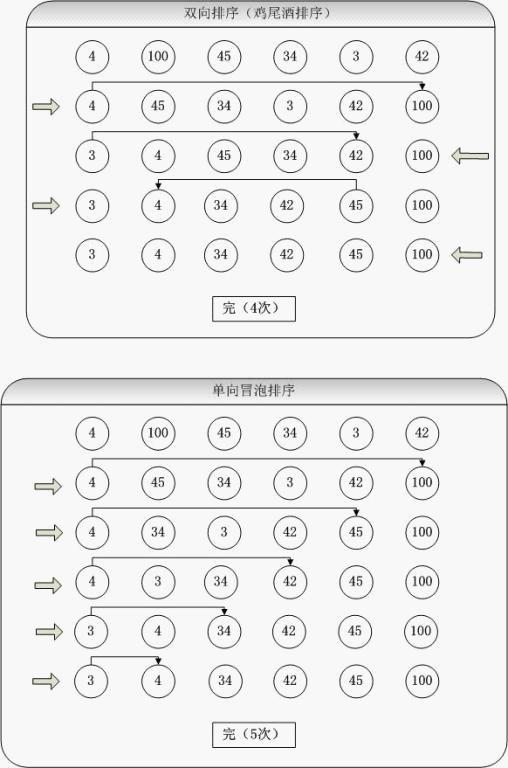## 4）算法代码

[cpp]  view plain copy
1. void CocktailSort(int *a,int nsize)
2. {
3.     int tail=nsize-1;
4.     for (int i=0;i<tail;)
5.     {
6.         for (int j=tail;j>i;--j) //第一轮，先将最小的数据排到前面
7.         {
8.             if (a[j]<a[j-1])
9.             {
10.                 int temp=a[j];
11.                 a[j]=a[j-1];
12.                 a[j-1]=temp;
13.             }
14.         }
15.         ++i;                    //原来i处数据已排好序，加1
16.         for (j=i;j<tail;++j)    //第二轮，将最大的数据排到后面
17.         {
18.             if (a[j]>a[j+1])
19.             {
20.                 int temp=a[j];
21.                 a[j]=a[j+1];
22.                 a[j+1]=temp;
23.             }
24.         }
25.         tail--;                 //原tail处数据也已排好序，将其减1
26.     }
27. }

## 5）考察点，重点和频度分析

鸡尾酒排序在博主印象中出现的频度也不高，用到它的算法题大题很少，选择填空出现的话多以双向冒泡排序的名称出现，注意注意时间空间复杂度，理解理解算法应该问题就不大了。

## 6）笔试面试例题

考点基本类似冒泡排序，请参考上一节

# 七、快速排序

恩，重头戏开始了，快速排序是各种笔试面试最爱考的排序算法之一，且排序思想在很多算法题里面被广泛使用。是需要重点掌握的排序算法。

## 1）算法简介

快速排序是由东尼·霍尔所发展的一种排序算法。其基本思想是基本思想是，通过一趟排序将待排记录分隔成独立的两部分，其中一部分记录的关键字均比另一部分的关键字小，则可分别对这两部分记录继续进行排序，以达到整个序列有序。

## 2）算法描述和分析

1、从数列中挑出一个元素，称为 "基准"（pivot），

2、重新排序数列，所有元素比基准值小的摆放在基准前面，所有元素比基准值大的摆在基准的后面（相同的数可以到任一边）。在这个分区退出之后，该基准就处于数列的中间位置。这个称为分区（partition）操作。

3、递归地（recursive）把小于基准值元素的子数列和大于基准值元素的子数列排序。

递归的最底部情形，是数列的大小是零或一，也就是永远都已经被排序好了。虽然一直递归下去，但是这个算法总会退出，因为在每次的迭代（iteration）中，它至少会把一个元素摆到它最后的位置去。

function quicksort(q)

var list less, pivotList, greater

if length(q) ≤ 1 {

return q

} else {

select a pivot value pivot from q

for each x in q except the pivot element

if x < pivot then add x to less

if x ≥ pivot then add x to greater

return concatenate(quicksort(less), pivotList, quicksort(greater))

}

 最差时间复杂度 O(n^2) 最优时间复杂度 O(n log n) 平均时间复杂度 O(n log n) 最差空间复杂度 根据实现的方式不同而不同

## 3）算法图解、flash演示、视频演示

### 图解：

快速排序会递归地进行很多轮，其中每一轮称之为快排的partition算法，即上述算法描述中的第2步，非常重要，且在各种笔试面试中用到该思想的算法题层出不穷，下图为第一轮的partition算法的一个示例。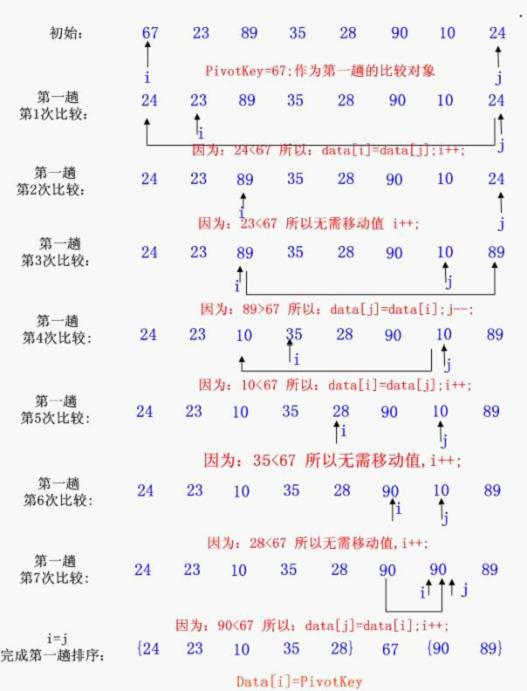## 4）算法代码

事实上，这个地方需要提一下的是，快排有很多种版本。例如，我们“基准数”的选择方法不同就有不同的版本，但重要的是快排的思想，我们熟练掌握一种版本，在最后的笔试面试中也够用了，我这里罗列几种最有名的版本C代码。

### 1、版本一

[cpp]  view plain copy
1. /**************************************************
2.   函数功能：对数组快速排序
3.   函数参数：指向整型数组arr的首指针arr；
4.             整型变量left和right左右边界的下标
5.   函数返回值：空
6. /**************************************************/
7. void QuickSort(int *arr, int left, int right)
8. {
9.   int i,j;
10.   if(left<right)
11.   {
12.     i=left;j=right;
13.     arr=arr[i]; //准备以本次最左边的元素值为标准进行划分，先保存其值
14.     do
15.     {
16.       while(arr[j]>arr && i<j)
17.         j--;        //从右向左找第1个小于标准值的位置j
18.       if(i<j)                               //找到了，位置为j
19.       {
20.         arr[i] = arr[j];
21.         i++;
22.       }           //将第j个元素置于左端并重置i
23.       while(arr[i]<arr && i<j)
24.         i++;      //从左向右找第1个大于标准值的位置i
25.       if(i<j)                       //找到了，位置为i
26.       {
27.         arr[j] = arr[i];
28.         j--;
29.       }           //将第i个元素置于右端并重置j
30.     }while(i!=j);
31.     arr[i] = arr;         //将标准值放入它的最终位置,本次划分结束
32.     quicksort(arr, left, i-1);     //对标准值左半部递归调用本函数
33.     quicksort(arr, i+1, right);    //对标准值右半部递归调用本函数
34.   }
35. }

### 2、版本二

[cpp]  view plain copy
1. //使用引用，完成两数交换
2. void Swap(int& a , int& b)
3. {
4.  int temp = a;
5.  a = b;
6.  b = temp;
7. }
8. //取区间内随机数的函数
9. int Rand(int low, int high)
10. {
11.  int size = hgh - low + 1;
12.  return  low + rand()%size;
13. }
14.     //快排的partition算法，这里的基准数是随机选取的
15. int RandPartition(int* data, int low , int high)
16. {
17.  swap(data[rand(low,high)], data[low]);//
18.  int key = data[low];
19.  int i = low;
20.
21.  for(int j=low+1; j<=high; j++)
22.  {
23.   if(data[j]<=key)
24.   {
25.    i = i+1;
26.    swap(data[i], data[j]);
27.   }
28.  }
29.  swap(data[i],data[low]);
30.  return i;
31. }
32. //递归完成快速排序
33. void QuickSort(int* data, int low, int high)
34. {
35.  if(low<high)
36.  {
37.   int k = RandPartition(data,low,high);
38.   QuickSort(data,low,k-1);
39.   QuickSort(data,k+1,high);
40.  }
41. }

## 5）考察点，重点和频度分析

完全考察快排算法本身的题目，多出现在选择填空，基本是关于时间空间复杂度的讨论，最好最坏的情形交换次数等等。倒是快排的partition算法需要特别注意！频度极高地被使用在各种算法大题中！详见下小节列举的面试小题。

## 6）笔试面试例题

这里要重点强调的是快排的partition算法，博主当年面试的时候就遇到过数道用该思路的算法题，举几道如下：

### 例题1、

当然，博主也知道这题可以建大小为k的大顶堆，然后用堆的方法解决。

但是这个题目可也以仿照快速排序，运用partition函数进行求解不过我们完整的快速排序分割后要递归地对前后两段继续进行分割，而这里我们需要做的是判定分割的位置，然后再确定对前段还是后段进行分割，所以只对单侧分割即可。代码如下：

[cpp]  view plain copy
1. void GetLeastNumbers_by_partition(int* input, int n, int* output, int k)
2. {
3.     if(input == NULL || output == NULL || k > n || n <= 0 || k <= 0)
4.         return;
5.     int start = 0;
6.     int end = n - 1;
7.     int index = Partition(input, n, start, end);
8.     while(index != k - 1)
9.     {
10.         if(index > k - 1)
11.         {
12.             end = index - 1;
13.             index = Partition(input, n, start, end);
14.         }
15.         else
16.         {
17.             start = index + 1;
18.             index = Partition(input, n, start, end);
19.         }
20.     }
21.     for(int i = 0; i < k; ++i)
22.         output[i] = input[i];
23. }

### 例题2、

当然，这道题很多人都见过，而且最通用的一种解法是数对对消的思路。这里只是再给大家提供一种思路，快排partition的方法在很多地方都能使用，比如这题。我们也可以选择合适的判定条件进行递归。代码如下：

[cpp]  view plain copy
1. bool g_bInputInvalid = false;
2. bool CheckInvalidArray(int* numbers, int length)
3. {
4.     g_bInputInvalid = false;
5.     if(numbers == NULL && length <= 0)
6.         g_bInputInvalid = true;
7.     return g_bInputInvalid;
8. }
9. bool CheckMoreThanHalf(int* numbers, int length, int number)
10. {
11.     int times = 0;
12.     for(int i = 0; i < length; ++i)
13.     {
14.         if(numbers[i] == number)
15.             times++;
16.     }
17.     bool isMoreThanHalf = true;
18.     if(times * 2 <= length)
19.     {
20.         g_bInputInvalid = true;
21.         isMoreThanHalf = false;
22.     }
23.     return isMoreThanHalf;
24. }
25. int MoreThanHalfNum_Solution1(int* numbers, int length)
26. {
27.     if(CheckInvalidArray(numbers, length))
28.         return 0;
29.     int middle = length >> 1;
30.     int start = 0;
31.     int end = length - 1;
32.     int index = Partition(numbers, length, start, end);
33.     while(index != middle)
34.     {
35.         if(index > middle)
36.         {
37.             end = index - 1;
38.             index = Partition(numbers, length, start, end);
39.         }
40.         else
41.         {
42.             start = index + 1;
43.             index = Partition(numbers, length, start, end);
44.         }
45.     }
46.     int result = numbers[middle];
47.     if(!CheckMoreThanHalf(numbers, length, result))
48.         result = 0;
49.     return result;
50. }

### 例题3、

这题可能大家都能想到的方法是：设置首尾两个指针，首指针向后移动寻找大写字母，尾指针向前移动需找小写字母，找到后都停下，交换。之后继续移动，直至相遇。这种方法在这里我就不做讨论写代码了。

但是这题也可以采用类似快排的partition。这里使用从左往后扫描的方式。字符串在调整的过程中可以分成两个部分：已排好的小写字母部分、待调整的剩余部分。用两个指针i和j，其中i指向待调整的剩余部分的第一个元素，用j指针遍历待调整的部分。当j指向一个小写字母时，交换i和j所指的元素。向前移动i、j，直到字符串末尾。代码如下：

[cpp]  view plain copy
1. #include <iostream>
2. using namespace std;
3. void Proc( char *str )
4. {
5. int i = 0;
6. int j = 0;
7. //移动指针i, 使其指向第一个大写字母
8. while( str[i] != '\0' && str[i] >= 'a' && str[i] <= 'z' ) i++;
9. if( str[i] != '\0' )
10. {
11. //指针j遍历未处理的部分，找到第一个小写字母
12. for( j=i; str[j] != '\0'; j++ )
13. {
14. if( str[j] >= 'a' && str[j] <= 'z' )
15. {
16. char tmp = str[i];
17. str[i] = str[j];
18. str[j] = tmp;
19. i++;
20. }
21. }
22. }
23. }
24. int main()
25. {
26. char data[] = "SONGjianGoodBest";
27. Proc( data );
28. return 0;
29. }

# 八、堆排序

不得不说，堆排序太容易出现了，选择填空问答算法大题都会出现。建堆的过程，堆调整的过程，这些过程的时间复杂度，空间复杂度，以及如何应用在海量数据Top K问题中等等，都是需要重点掌握的。

## 1）算法简介

堆排序（Heapsort）是指利用堆这种数据结构所设计的一种排序算法。堆积是一个近似完全二叉树的结构，并同时满足堆积的性质：即子结点的键值或索引总是小于（或者大于）它的父节点。

## 2）算法描述

我们这里介绍几个问题，一步步推到堆排序的算法。

### 1、什么是堆？

我们这里提到的堆一般都指的是二叉堆，它满足二个特性：

1---父结点的键值总是大于或等于（小于或等于）任何一个子节点的键值。

2---每个结点的左子树和右子树都是一个二叉堆（都是最大堆或最小堆）。

如下为一个最小堆(父结点的键值总是小于任何一个子节点的键值)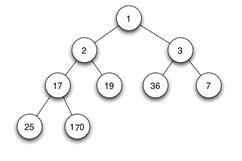这是为了保持堆的特性而做的一个操作。对某一个节点为根的子树做堆调整，其实就是将该根节点进行“下沉”操作(具体是通过和子节点交换完成的)，一直下沉到合适的位置，使得刚才的子树满足堆的性质

例如对最大堆的堆调整我们会这么做：

1、在对应的数组元素A[i], 左孩子A[LEFT(i)], 和右孩子A[RIGHT(i)]中找到最大的那一个，将其下标存储在largest中。

2、如果A[i]已经就是最大的元素，则程序直接结束。

3、否则，i的某个子结点为最大的元素，将A[largest]与A[i]交换。

4、再从交换的子节点开始，重复1,2,3步，直至叶子节点，算完成一次堆调整。

这里需要提一下的是，一般做一次堆调整的时间复杂度为log(n)

如下为我们对4为根节点的子树做一次堆调整的示意图，可帮我们理解。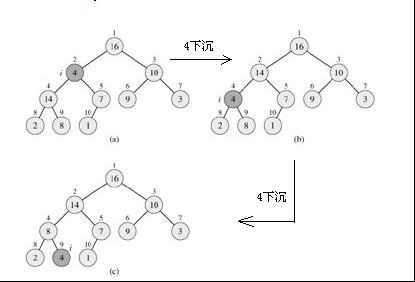### 3、如何建堆

建堆是一个通过不断的堆调整，使得整个二叉树中的数满足堆性质的操作。在数组中的话，我们一般从下标为n/2的数开始做堆调整，一直到下标为0的数(因为下标大于n/2的数都是叶子节点，其子树已经满足堆的性质了)。下图为其一个图示：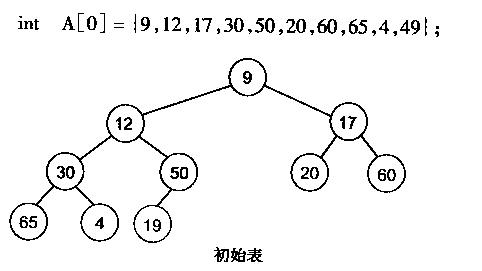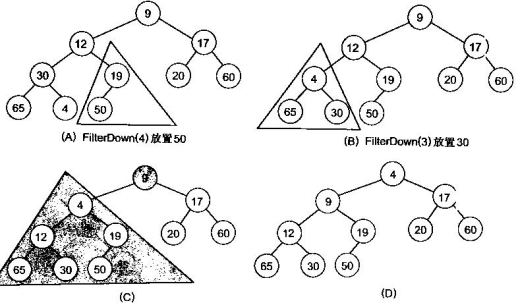很明显，对叶子结点来说，可以认为它已经是一个合法的堆了即2060， 65， 4， 49都分别是一个合法的堆。只要从A=50开始向下调整就可以了。然后再取A=30A = 17A = 12A = 9分别作一次向下调整操作就可以了。

### 4、如何进行堆排序

堆排序是在上述3中对数组建堆的操作之后完成的。

数组储存成堆的形式之后，第一次将AA[n - 1]交换，再对A[0n-2]重新恢复堆。第二次将AA[n-2]交换，再对A[0n-3]重新恢复堆，重复这样的操作直到AA交换。由于每次都是将最小的数据并入到后面的有序区间，故操作完成后整个数组就有序了。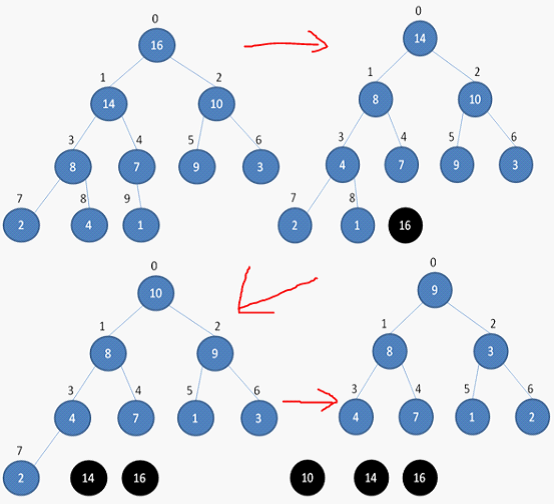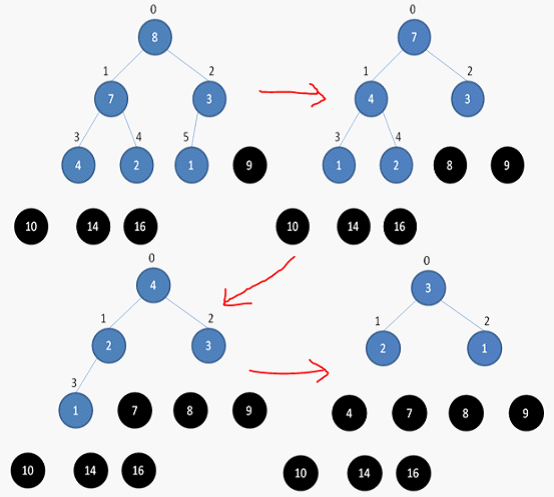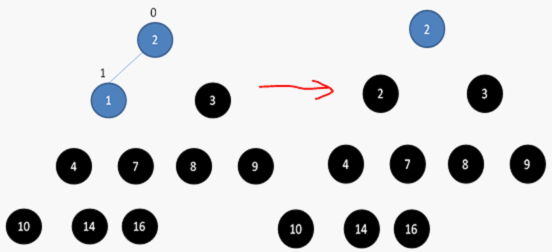最差时间复杂度 O(n log n) 最优时间复杂度 O(n log n) 平均时间复杂度 O(n log n) 最差空间复杂度 O(n)

略，见上一节。

## 4）算法代码

[cpp]  view plain copy
1. #include <cstdio>
2. #include <cstdlib>
3. #include <cmath>
4. using namespace std;
5.
6. int parent(int);
7. int left(int);
8. int right(int);
10. void BuildHeap(int [], int);
11. void print(int [], int);
12. void HeapSort(int [], int);
13.
14. /*返回父节点*/
15. int parent(int i)
16. {
17.     return (int)floor((i - 1) / 2);
18. }
19.
20. /*返回左孩子节点*/
21. int left(int i)
22. {
23.     return (2 * i + 1);
24. }
25.
26. /*返回右孩子节点*/
27. int right(int i)
28. {
29.     return (2 * i + 2);
30. }
31.
32. /*对以某一节点为根的子树做堆调整(保证最大堆性质)*/
33. void HeapAdjust(int A[], int i, int heap_size)
34. {
35.     int l = left(i);
36.     int r = right(i);
37.     int largest;
38.     int temp;
39.     if(l < heap_size && A[l] > A[i])
40.     {
41.         largest = l;
42.     }
43.     else
44.     {
45.         largest = i;
46.     }
47.     if(r < heap_size && A[r] > A[largest])
48.     {
49.         largest = r;
50.     }
51.     if(largest != i)
52.     {
53.         temp = A[i];
54.         A[i] = A[largest];
55.         A[largest] = temp;
57.     }
58. }
59.
60. /*建立最大堆*/
61. void BuildHeap(int A[]，int heap_size)
62. {
63.     for(int i = (heap_size-2)/2; i >= 0; i--)
64.     {
66.     }
67. }
68.
69. /*输出结果*/
70. void print(int A[]， int heap_size)
71. {
72.     for(int i = 0; i < heap_size;i++)
73.     {
74.         printf("%d ", A[i]);
75.     }
76.     printf("\n");
77. }
78.
79. /*堆排序*/
80. void HeapSort(int A[], int heap_size)
81. {
82.     BuildHeap(A, heap_size);
83.     int temp;
84.     for(int i = heap_size - 1; i >= 0; i--)
85.     {
86.         temp = A;
87.         A = A[i];
88.         A[i] = temp;
90.     }
91.     print(A, heap_size);
92. }
93.
94. /*测试，对给定数组做堆排序*/
95. int main(int argc, char* argv[])
96. {
97.     const int heap_size = 13;
98.     int A[] = {19, 1, 10, 14, 16, 4, 7, 9, 3, 2, 8, 5, 11};
99.     HeapSort(A, heap_size);
100.     system("pause");
101.     return 0;
102. }

## 5）考察点，重点和频度分析

堆排序相关的考察太多了，选择填空问答算法大题都会出现。建堆的过程，堆调整的过程，这些过程的时间复杂度，空间复杂度，需要比较交换多少次，以及如何应用在海量数据Top K问题中等等。堆又是一种很好做调整的结构，在算法题里面使用频度很高。

## 6）笔试面试题

### 例题1、

典型的Top K问题，用堆是最典型的思路。建10000个数的小顶堆，然后将10亿个数依次读取，大于堆顶，则替换堆顶，做一次堆调整。结束之后，小顶堆中存放的数即为所求。代码如下(为了方便，这里直接使用了STL容器)

[cpp]  view plain copy
1. #include "stdafx.h"
2. #include <vector>
3. #include <iostream>
4. #include <algorithm>
5. #include <functional> // for greater<>
6. using namespace std;
7. int _tmain(int argc, _TCHAR* argv[])
8. {
9.   vector<float> bigs(10000,0);
10.   vector<float>::iterator it;
11.   // Init vector data
12.   for (it = bigs.begin(); it != bigs.end(); it++)
13.   {
14.     *it = (float)rand()/7; // random values;
15.   }
16.   cout << bigs.size() << endl;
17.   make_heap(bigs.begin(),bigs.end(), greater<float>()); // The first one is the smallest one!
18.   float ff;
19.   for (int i = 0; i < 1000000000; i++)
20.   {
21.     ff = (float) rand() / 7;
22.     if (ff > bigs.front()) // replace the first one ?
23.     {
24.       // set the smallest one to the end!
25.       pop_heap(bigs.begin(), bigs.end(), greater<float>());
26.       // remove the last/smallest one
27.       bigs.pop_back();
28.       // add to the last one
29.       bigs.push_back(ff);
30.       // mask heap again, the first one is still the smallest one
31.       push_heap(bigs.begin(),bigs.end(),greater<float>());
32.     }
33.   }
34.   // sort by ascent
35.   sort_heap(bigs.begin(), bigs.end(), greater<float>());
36.   return 0;
37. }

### 例题2、

使用大顶堆和小顶堆存储。

使用大顶堆存储较小的一半数字，使用小顶堆存储较大的一半数字。

插入数字时，在O(logn)时间内将该数字插入到对应的堆当中，并适当移动根节点以保持两个堆数字相等（或相差1）。

获取中数时，在O(1)时间内找到中数。

# 九、归并排序

## 1）算法简介

归并排序是建立在归并操作上的一种有效的排序算法。该算法是采用分治法（Divide and Conquer）的一个非常典型的应用。归并排序是一种稳定的排序方法。

将已有序的子序列合并，得到完全有序的序列；即先使每个子序列有序，再使子序列段间有序。若将两个有序表合并成一个有序表，称为2-路归并。

## 2）算法描述

归并排序具体算法描述如下(递归版本)：

1、Divide: 把长度为n的输入序列分成两个长度为n/2的子序列。

2、Conquer: 对这两个子序列分别采用归并排序。

3、Combine: 将两个排序好的子序列合并成一个最终的排序序列。

归并排序的效率是比较高的，设数列长为N将数列分开成小数列一共要logN步，每步都是一个合并有序数列的过程，时间复杂度可以记为O(N)，故一共为O(N*logN)。因为归并排序每次都是在相邻的数据中进行操作，所以归并排序在O(N*logN)的几种排序方法（快速排序，归并排序，希尔排序，堆排序）也是效率比较高的。

## 3）算法图解、flash演示、视频演示

### 图解：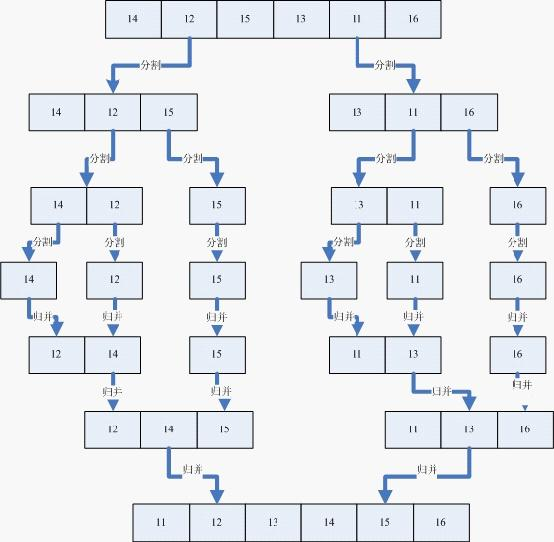## 4）算法代码

[cpp]  view plain copy
1. //将有二个有序数列a[first...mid]和a[mid...last]合并。
2. void MergeArray(int a[], int first, int mid, int last, int temp[])
3. {
4. int i = first, j = mid + 1;
5. int m = mid,   n = last;
6. int k = 0;
7. while (i <= m && j <= n)
8. {
9. if (a[i] <= a[j])
10. temp[k++] = a[i++];
11. else
12. temp[k++] = a[j++];
13. }
14. while (i <= m)
15. temp[k++] = a[i++];
16. while (j <= n)
17. temp[k++] = a[j++];
18. for (i = 0; i < k; i++)
19. a[first + i] = temp[i];
20. }
21. //递归地完成归并排序
22. void MergeSort(int a[], int first, int last, int temp[])
23. {
24. if (first < last)
25. {
26. int mid = (first + last) / 2;
27. mergesort(a, first, mid, temp);    //左边有序
28. mergesort(a, mid + 1, last, temp); //右边有序
29. mergearray(a, first, mid, last, temp); //再将二个有序数列合并
30. }
31. }

## 5）考察点、重点和频度分析

归并排序本身作为一种高效的排序算法，也是常会被问到的。尤其是归并排序体现的递归思路很重要，在递归的过程中可以完成很多事情，很多算法题也是使用的这个思路，可见下面7）部分的笔试面试算法题。

## 6）笔试面试题

### 例题1、

这题求解的其实就是一个逆序对。我们回想一下归并排序的过程：

归并排序是用分治思想，分治模式在每一层递归上有三个步骤：

分解：将n个元素分成个含n/2个元素的子序列。

解决：用合并排序法对两个子序列递归的排序。

合并：合并两个已排序的子序列已得到排序结果。

在归并排序算法中稍作修改，就可以在n log n的时间内求逆序对。

将数组A[1...size]，划分为A[1...mid] 和 A[mid+1...size].那么逆序对数的个数为 f(1, size) = f(1, mid) + f(mid+1, size) + s(1, mid, size)，这里s(1, mid, size)代表左值在[1---mid]中，右值在[mid+1, size]中的逆序对数。由于两个子序列本身都已经排序，所以查找起来非常方便。

[cpp]  view plain copy
1. #include<iostream>
2. #include<stdlib.h>
3. using namespace std;
4. void printArray(int arry[],int len)
5. {
6.     for(int i=0;i<len;i++)
7.         cout<<arry[i]<<" ";
8.     cout<<endl;
9. }
10. int MergeArray(int arry[],int start,int mid,int end,int temp[])//数组的归并操作
11. {
12.     //int leftLen=mid-start+1;//arry[start...mid]左半段长度
13.     //int rightLlen=end-mid;//arry[mid+1...end]右半段长度
14.     int i=mid;
15.     int j=end;
16.     int k=0;//临时数组末尾坐标
17.     int count=0;
18.     //设定两个指针ij分别指向两段有序数组的头元素，将小的那一个放入到临时数组中去。
19.     while(i>=start&&j>mid)
20.     {
21.         if(arry[i]>arry[j])
22.         {
23.             temp[k++]=arry[i--];//从临时数组的最后一个位置开始排序
24.             count+=j-mid;//因为arry[mid+1...j...end]是有序的，如果arry[i]>arry[j]，那么也大于arry[j]之前的元素，从a[mid+1...j]一共有j-(mid+1)+1=j-mid
25.
26.         }
27.         else
28.         {
29.             temp[k++]=arry[j--];
30.         }
31.     }
32.     cout<<"调用MergeArray时的count："<<count<<endl;
33.     while(i>=start)//表示前半段数组中还有元素未放入临时数组
34.     {
35.         temp[k++]=arry[i--];
36.     }
37.     while(j>mid)
38.     {
39.         temp[k++]=arry[j--];
40.     }
41.     //将临时数组中的元素写回到原数组当中去。
42.     for(i=0;i<k;i++)
43.         arry[end-i]=temp[i];
44.     printArray(arry,8);//输出进过一次归并以后的数组，用于理解整体过程
45.     return count;
46. }
47. int InversePairsCore(int arry[],int start,int end,int temp[])
48. {
49.     int inversions = 0;
50.     if(start<end)
51.     {
52.         int mid=(start+end)/2;
53.         inversions+=InversePairsCore(arry,start,mid,temp);//找左半段的逆序对数目
54.         inversions+=InversePairsCore(arry,mid+1,end,temp);//找右半段的逆序对数目
55.         inversions+=MergeArray(arry,start,mid,end,temp);//在找完左右半段逆序对以后两段数组有序，然后找两段之间的逆序对。最小的逆序段只有一个元素。
56.     }
57.     return inversions;
58. }
59. int InversePairs(int arry[],int len)
60. {
61.     int *temp=new int[len];
62.     int count=InversePairsCore(arry,0,len-1,temp);
63.     delete[] temp;
64.     return count;
65. }
66. void main()
67. {
68.     //int arry[]={7,5,6,4};
69.     int arry[]={1,3,7,8,2,4,6,5};
70.     int len=sizeof(arry)/sizeof(int);
71.     //printArray(arry,len);
72.     int count=InversePairs(arry,len);
73.     //printArray(arry,len);
74.     //cout<<count<<endl;
75.     system("pause");
76. }

### 例题2、

1hash映射：顺序读取10个文件，按照hash(query)%10的结果将query写入到另外10个文件（记为）中。这样新生成的文件每个的大小大约也1G（假设hash函数是随机的）。

2hash统计：找一台内存在2G左右的机器，依次对用hash_map(query, query_count)来统计每个query出现的次数。注：hash_map(query,query_count)是用来统计每个query的出现次数，不是存储他们的值，出现一次，则count+1

3堆/快速/归并排序：利用快速//归并排序按照出现次数进行排序。将排序好的query和对应的query_cout输出到文件中。这样得到了10个排好序的文件（记为）。对这10个文件进行归并排序（内排序与外排序相结合）。

### 例题3、

使用原地归并，能够让归并排序的空间复杂度降为O(1)，但是速度上会有一定程度的下降。代码如下：

[cpp]  view plain copy
1. #include<iostream>
2. #include<cmath>
3. #include<cstdlib>
4. #include<Windows.h>
5. using namespace std;
6. void insert_sort(int arr[],int n)
7. {
8. for(int i=1;i<n;++i)
9. {
10. int val=arr[i];
11. int j=i-1;
12. while(arr[j]>val&&j>=0)
13. {
14. arr[j+1]=arr[j];
15. --j;
16. }
17. arr[j+1]=val;
18. }
19. }
20. void aux_merge(int arr[],int n,int m,int aux[])
21. {
22. for(int i=0;i<m;++i)
23. swap(aux[i],arr[n+i]);
24. int p=n-1,q=m-1;
25. int dst=n+m-1;
26. for(int i=0;i<n+m;++i)
27. {
28. if(p>=0)
29. {
30. if(q>=0)
31. {
32. if(arr[p]>aux[q])
33. {
34. swap(arr[p],arr[dst]);
35. p--;
36. }
37. else
38. {
39. swap(aux[q],arr[dst]);
40. q--;
41. }
42. }
43. else
44. break;
45. }
46. else
47. {
48. swap(aux[q],arr[dst]);
49. q--;
50. }
51. dst--;
52. }
53. }
54. void local_merge(int arr[],int n)
55. {
56. int m=sqrt((float)n);
57. int k=n/m;
58. for(int i=0;i<m;++i)
59. swap(arr[k*m-m+i],arr[n/2/m*m+i]);
60. for(int i=0;i<k-2;++i)
61. {
62. int index=i;
63. for(int j=i+1;j<k-1;++j)
64. if(arr[j*m]<arr[index*m])
65. index=j;
66. if(index!=i)
67. for(int j=0;j<m;++j)
68. swap(arr[i*m+j],arr[index*m+j]);
69. }
70. for(int i=0;i<k-2;++i)
71. aux_merge(arr+i*m,m,m,arr+(k-1)*m);
72. int s=n%m+m;
73. insert_sort(arr+(n-2*s),2*s);
74. aux_merge(arr,n-2*s,s,arr+(k-1)*m);
75. insert_sort(arr+(k-1)*m,s);
76. }
77. void local_merge_sort(int arr[],int n)
78. {
79. if(n<=1)
80. return;
81. if(n<=10)
82. {
83. insert_sort(arr,n);
84. return;
85. }
86. local_merge_sort(arr,n/2);
87. local_merge_sort(arr+n/2,n-n/2);
88. local_merge(arr,n);
89. }
90. void merge_sort(int arr[],int temp[],int n)
91. {
92. if(n<=1)
93. return;
94. if(n<=10)
95. {
96. insert_sort(arr,n);
97. return;
98. }
99. merge_sort(arr,temp,n/2);
100. merge_sort(arr+n/2,temp,n-n/2);
101. for(int i=0;i<n/2;++i)
102. temp[i]=arr[i];
103. for(int i=n/2;i<n;++i)
104. temp[n+n/2-i-1]=arr[i];
105. int left=0,right=n-1;
106. for(int i=0;i<n;++i)
107. if(temp[left]<temp[right])
108. arr[i]=temp[left++];
109. else
110. arr[i]=temp[right--];
111. }
112. const int n=2000000;
113. int arr1[n],arr2[n];
114. int temp[n];
115. int main()
116. {
117. for(int i=0;i<n;++i)
118. arr1[i]=arr2[i]=rand();
119. int begin=GetTickCount();
120. merge_sort(arr1,temp,n);
121. cout<<GetTickCount()-begin<<endl;
122. begin=GetTickCount();
123. local_merge_sort(arr2,n);
124. cout<<GetTickCount()-begin<<endl;
125. for(int i=0;i<n;++i)
126. if(arr1[i]!=arr2[i])
127. cout<<"ERROR"<<endl;
128. system("pause");
129. }

# 十、桶排序

## 1）算法简介

桶排序 (Bucket sort)或所谓的箱排序，是一个排序算法，工作的原理是将数组分到有限数量的桶子里。每个桶子再个别排序（有可能再使用别的排序算法或是以递归方式继续使用桶排序进行排序）

桶排序是稳定的，且在大多数情况下常见排序里最快的一种,比快排还要快，缺点是非常耗空间,基本上是最耗空间的一种排序算法，而且只能在某些情形下使用。

## 2）算法描述和分析

桶排序具体算法描述如下：

1、设置一个定量的数组当作空桶子。

2、寻访串行，并且把项目一个一个放到对应的桶子去。

3、对每个不是空的桶子进行排序。

4、从不是空的桶子里把项目再放回原来的串行中。

桶排序最好情况下使用线性时间O(n)，很显然桶排序的时间复杂度，取决与对各个桶之间数据进行排序的时间复杂度，因为 其它部分的时间复杂度都为O(n);很显然，桶划分的越小，各个桶之间的数据越少，排 序所用的时间也会越少。但相应的空间消耗就会增大。

可以证明，即使选用插入排序作为桶内排序的方法，桶排序的平均时间复杂度为线性。 具体证明，请参考算法导论。其空间复杂度也为线性。

## 3）算法图解、flash演示、视频演示

### 图解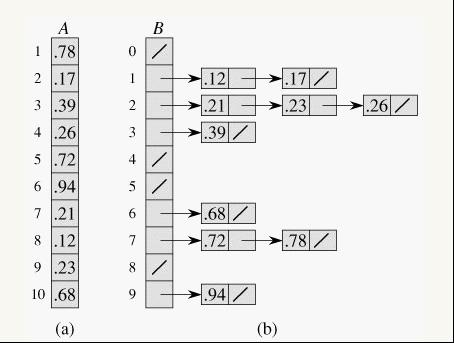## 4）算法代码

[cpp]  view plain copy
1. #include <time.h>
2. #include <iostream>
3. #include <iomanip>
4. using namespace  std;
5.
6. /*initial arr*/
7. void InitialArr(double *arr,int n)
8. {
9. srand((unsigned)time(NULL));
10. for (int i = 0; i<n;i++)
11. {
12. arr[i] = rand()/double(RAND_MAX+1);   //(0.1)
13. }
14. }
15.
16. /* print arr*/
17. void PrintArr(double *arr,int n)
18. {
19. for (int i = 0;i < n; i++)
20. {
21. cout<<setw(15)<<arr[i];
22. if ((i+1)%5 == 0 || i ==  n-1)
23. {
24. cout<<endl;
25. }
26. }
27. }
28.
29. void BucketSort(double * arr,int n)
30. {
31. double **bucket = new double*;
32. for (int i = 0;i<10;i++)
33. {
34. bucket[i] = new double[n];
35. }
36. int count = {0};
37. for (int i = 0 ; i < n ; i++)
38. {
39. double temp = arr[i];
40. int flag = (int)(arr[i]*10); //flag标识小树的第一位
41. bucket[flag][count[flag]] = temp; //用二维数组的每个向量来存放小树第一位相同的数据
42. int j = count[flag]++;
43.
44. /* 利用插入排序对每一行进行排序 */
45. for(;j > 0 && temp < bucket[flag][j - 1]; --j)
46. {
47. bucket[flag][j] = bucket[flag][j-1];
48. }
49. bucket[flag][j] =temp;
50. }
51.
52. /* 所有数据重新链接 */
53. int k=0;
54. for (int i = 0 ; i < 10 ; i++)
55. {
56. for (int j = 0 ; j< count[i];j++)
57. {
58. arr[k] = bucket[i][j];
59. k++;
60. }
61. }
62. for (int i = 0 ; i<10 ;i++)
63. {
64. delete bucket[i];
65. bucket[i] =NULL;
66. }
67. delete []bucket;
68. bucket = NULL;
69. }
70.
71. void main()
72. {
73. double *arr=new double;
74. InitialArr(arr, 10);
75. BucketSort(arr, 10);
76. PrintArr(arr,10);
77. delete [] arr;
78. }

## 5）考察点、重点和频度分析

桶排序是一种很巧妙的排序方法，在处理密集型数排序的时候有比较好的效果(主要是这种情况下空间复杂度不高)，其思想也可用在很多算法题上，详见后续笔试面试算法例题

## 6）笔试面试题

### 例题1、

对500W数据排序，如果基于比较的先进排序，平均比较次数为O(5000000*log5000000)≈1.112亿。但是我们发现，这些数据都有特殊的条件：  100=<score<=900。那么我们就可以考虑桶排序这样一个投机取巧的办法、让其在毫秒级别就完成500万排序。

创建801(900-100)个桶。将每个考生的分数丢进f(score)=score-100的桶中。这个过程从头到尾遍历一遍数据只需要500W次。然后根据桶号大小依次将桶中数值输出，即可以得到一个有序的序列。而且可以很容易的得到100分有***人，501分有***人。

实际上，桶排序对数据的条件有特殊要求，如果上面的分数不是从100-900，而是从0-2亿，那么分配2亿个桶显然是不可能的。所以桶排序有其局限性，适合元素值集合并不大的情况。

### 例题2、

分析： 既然要找中位数，很简单就是排序的想法。那么基于字节的桶排序是一个可行的方法。

思想：将整型的每1byte作为一个关键字，也就是说一个整形可以拆成4keys，而且最高位的keys越大，整数越大。如果高位keys相同，则比较次高位的keys。整个比较过程类似于字符串的字典序。按以下步骤实施：

1把10G整数每2G读入一次内存，然后一次遍历这536,870,912即（1024*1024*1024*2 /4个数据。每个数据用位运算">>"取出最高8(31-24)。这8bits(0-255)最多表示255个桶，那么可以根据8bit的值来确定丢入第几个桶。最后把每个桶写入一个磁盘文件中，同时在内存中统计每个桶内数据的数量，自然这个数量只需要255个整形空间即可。
2继续以内存中的整数的次高8bit进行桶排序(23-16)。过程和第一步相同，也是255个桶。
3一直下去，直到最低字节(7-0bit)的桶排序结束。我相信这个时候完全可以在内存中使用一次快排就可以了。

### 例题3、

典型的最大间隙问题。

要求线性时间算法。需要使用桶排序。桶排序的平均时间复发度是O(N).如果桶排序的数据分布不均匀，假设都分配到同一个桶中，最坏情况下的时间复杂度将变为O(N^2).

桶排序: 最关键的建桶，如果桶设计得不好的话桶排序是几乎没有作用的。通常情况下，上下界有两种取法，第一种是取一个10^n或者是2^n的数，方便实现。另一种是取数列的最大值和最小值然后均分作桶。

对于这个题，最关键的一步是：由抽屉原理知：最大差值M>= (Max(V[n])-Min(V[n]))/(n-1)所以，假如以(Max(V[n])-Min(V[n]))/(n-1)为桶宽的话，答案一定不是属于同一个桶的两元素之差。因此，这样建桶，每次只保留桶里面的最大值和最小值即可。

[cpp]  view plain copy
1. //距离平均值为offset = (arrayMax - arrayMin) / (n - 1), 则距离最大的数必然大于这个值
2. //每个桶只要记住桶中的最大值和最小值，依次比较上一个桶的最大值与下一个桶的最小值的差值，找最大的即可.
3. #include <iostream>
4. #define MAXSIZE 100    //实数的个数
5. #define MAXNUM 32767
6. using namespace std;
7. struct Barrel
8. {
9.  double min;   //桶中最小的数
10.  double max;   //桶中最大的数
11.  bool flag;   //标记桶中有数
12. };
13. int BarrelOperation(double* array, int n)
14. {
15.  Barrel barrel[MAXSIZE];  //实际使用的桶
16.  int nBarrel = 0;  //实际使用桶的个数
17.  Barrel tmp[MAXSIZE];   //临时桶，用于暂存数据
18.  double arrayMax = -MAXNUM, arrayMin = MAXNUM;
19.  for(int i = 0; i < n; i++) {
20.   if(array[i] > arrayMax)
21.    arrayMax = array[i];
22.   if(array[i] < arrayMin)
23.    arrayMin = array[i];
24.  }
25.  double offset = (arrayMax - arrayMin) / (n - 1);  //所有数的平均间隔
26.  //对桶进行初始化
27.  for(i = 0; i < n; i++) {
28.   tmp[i].flag = false;
29.   tmp[i].max = arrayMin;
30.   tmp[i].min = arrayMax;
31.  }
32.  //对数据进行分桶
33.  for(i = 0; i < n; i++) {
34.   int pos = (int)((array[i] - arrayMin) / offset);
35.   if(!tmp[pos].flag) {
36.    tmp[pos].max = tmp[pos].min = array[i];
37.    tmp[pos].flag = true;
38.   } else {
39.    if(array[i] > tmp[pos].max)
40.     tmp[pos].max = array[i];
41.    if(array[i] < tmp[pos].min)
42.     tmp[pos].min = array[i];
43.   }
44.  }
45.  for(i = 0; i <= n; i++) {
46.   if(tmp[i].flag)
47.    barrel[nBarrel++] = tmp[i];
48.  }
49.  int maxOffset = 0.0;
50.  for(i = 0; i < nBarrel - 1; i++) {
51.   if((barrel[i+1].min - barrel[i].max) > maxOffset)
52.    maxOffset = barrel[i+1].min - barrel[i].max;
53.  }
54.  return maxOffset;
55. }
56. int main()
57. {
58.  double array[MAXSIZE] = {1, 8, 6, 11, 7, 13, 16, 5};  //所需处理的数据
59.  int n = 8; //数的个数
60.  //double array[MAXSIZE] = {8, 6, 11};
61.  //int n = 3;
62.  int maxOffset = BarrelOperation(array, n);
63.  cout << maxOffset << endl;
64.  return 0;
65. }

# 十一、计数排序

## 1）算法简介

计数排序(Counting sort)是一种稳定的排序算法。计数排序使用一个额外的数组C，其中第i个元素是待排序数组A中值等于i的元素的个数。然后根据数组C来将A中的元素排到正确的位置只能对整数进行排序

## 2）算法描述和分析

算法的步骤如下：

1、找出待排序的数组中最大和最小的元素

2、统计数组中每个值为i的元素出现的次数，存入数组C的第i项

3、对所有的计数累加（从C中的第一个元素开始，每一项和前一项相加）

4、反向填充目标数组：将每个元素i放在新数组的第C(i)项，每放一个元素就将C(i)减去1

当输入的元素是n 0k之间的整数时，它的运行时间是 O(n + k)。计数排序不是比较排序，排序的速度快于任何比较排序算法。

由于用来计数的数组C的长度取决于待排序数组中数据的范围（等于待排序数组的最大值与最小值的差加上1），这使得计数排序对于数据范围很大的数组，需要大量时间和内存。例如：计数排序是用来排序0100之间的数字的最好的算法，但是它不适合按字母顺序排序人名。但是，计数排序可以用在基数排序中的算法来排序数据范围很大的数组。

## 3）算法图解、flash演示、视频演示

### 图解：

我们使用计数排序对一个乱序的整数数组进行排序。

首先创建一个临时数组（长度为输入数据的最大间隔），对于每一个输入数组的整数k，我们在临时数组的第k位置"1"如下图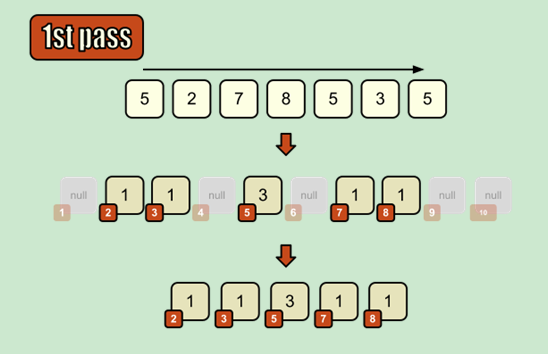上图中，第一行表示输入数据，第二行表示创建的临时数据，临时数组的下标代表输入数据的某一个值，临时数组的值表示输入数据中某一个值的数量。

如果输入数据中有重复的数值，那么我们增加临时数组相应的值（比如上图中53个，所以小标为5的数组的值是3）。在“初始化”临时数组以后，我们就得到了一个排序好的输入数据。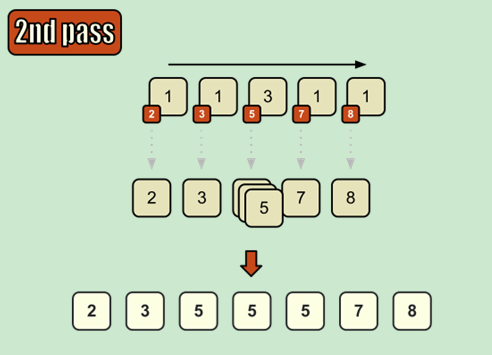我们顺序遍历这个数组，将下标解释成数据， 将该位置的值表示该数据的重复数量，记得得到一个排序好的数组。

### 视频：

前面的flash已经能够清晰地表示出整个计数排序的过程了，这里就不推荐视频了

## 4）算法代码

[cpp]  view plain copy
1. #include <stdlib.h>
2. #include <string.h>
3. #include <stdio.h>
4. /**************************************************************
5.  功能：计数排序。
6.  参数： data ： 要排序的数组
7.         size ：数组元素的个数
8.         k   ：数组中元素数组最大值 +1 （这个需要+1）
9.  返回值： 成功0；失败-1.
10.  *************************************************************/
11. int ctsort(int *data, int size, int k)
12. {
13.     int * counts = NULL,/*计数数组*/
14.         * temp = NULL;/*保存排序后的数组*/
15.     int i = 0;
16.     /*申请数组空间*/
17.     if ((counts = (int *) malloc( k * sizeof(int))) == NULL)
18.         return -1;
19.     if ((temp = (int *) malloc( k * sizeof(int))) == NULL)
20.         return -1;
21.     /*初始化计数数组*/
22.     for (i = 0; i < k; i ++)
23.         counts[i] = 0;
24.     /*数组中出现的元素，及出现次数记录*/
25.     for(i = 0; i < size; i++)
26.         counts[data[i]] += 1;
27.     /*调整元素计数中，加上前一个数*/
28.     for (i = 1; i < k; i++)
29.         counts[i] += counts[i - 1];
30.     /*使用计数数组中的记录数值，来进行排序，排序后保存的temp*/
31.     for (i = size -1; i >= 0; i --){
32.         temp[counts[data[i]] - 1] = data[i];
33.         counts[data[i]] -= 1;
34.     }
35.
36.     memcpy(data,temp,size * sizeof(int));
37.     free(counts);
38.     free(temp);
39.     return 0;
40. }
41. int main()
42. {
43.     int a = {2,0,2,1,4,6,7,4};
44.     int max = a,
45.         i = 0;
46.     /*获得数组中中的数值*/
47.     for ( i = 1; i < 8; i++){
48.         if (a[i] > max)
49.             max = a[i];
50.     }
51.     ctsort(a,8,max+1);
52.     for (i = 0;i < 8;i ++)
53.         printf("%d\n",a[i]);
54. }

## 6）笔试面试题

### 例题1、

够典型的计数排序吧，年龄的区间也就那么大，代码就不上了，请参照上述参照计数排序算法。

# 十二、基数排序

## 1）算法简介

基数排序是一种非比较型整数排序算法，其原理是将整数按位数切割成不同的数字，然后按每个位数分别比较。由于整数也可以表达字符串（比如名字或日期）和特定格式的浮点数，所以基数排序也不是只能使用于整数。基数排序的发明可以追溯到1887年赫尔曼·何乐礼在打孔卡片制表机（Tabulation Machine）上的贡献。

## 2）算法描述和分析

整个算法过程描述如下：

1、将所有待比较数值（正整数）统一为同样的数位长度，数位较短的数前面补零。

2、从最低位开始，依次进行一次排序。

3、这样从最低位排序一直到最高位排序完成以后, 数列就变成一个有序序列。

基数排序的时间复杂度是 O(kn)，其中n是排序元素个数，k是数字位数。

注意这不是说这个时间复杂度一定优于O(n·log(n))，因为k的大小一般会受到n的影响。 以排序n个不同整数来举例，假定这些整数以B为底，这样每位数都有B个不同的数字，k就一定不小于logB(n)。由于有B个不同的数字，所以就需要B个不同的桶，在每一轮比较的时候都需要平均n·log2(B) 次比较来把整数放到合适的桶中去，所以就有：

k 大于或等于 logB(n)

每一轮(平均)需要 n·log2(B) 次比较

T ≥ logB(n)·n·log2(B) = log2(n)·logB(2)·n·log2(B) = log2(n)·n·logB(2)·log2(B) = n·log2(n)

所以和比较排序相似，基数排序需要的比较次数：T ≥ n·log2(n)。 故其时间复杂度为 Ω(n·log2(n)) = Ω(n·log n)

## 3）算法图解、flash演示、视频演示

### 图解：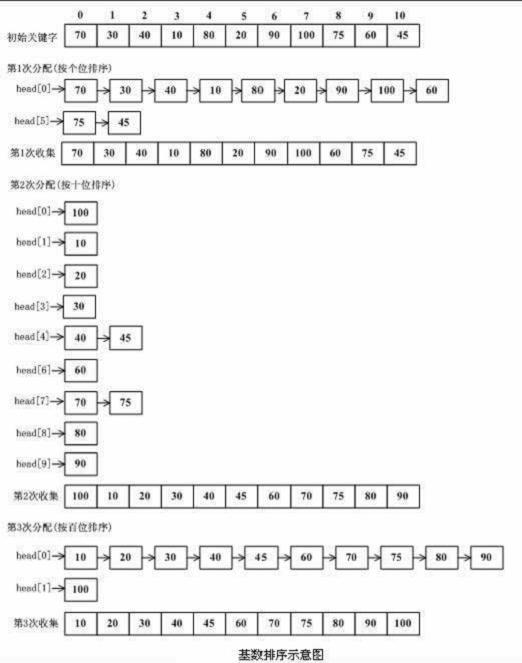## 4）算法代码

[cpp]  view plain copy
1. #include <stdio.h>
2. #include <stdlib.h>
4.     int temp = {0};
5.     int order = {0};
6.
7.     int n = 1;
8.     while(n <= 10) {
9.
10.         int i;
11.         for(i = 0; i < 10; i++) {
12.             int lsd = ((data[i] / n) % 10);
13.             temp[lsd][order[lsd]] = data[i];
14.             order[lsd]++;
15.         }
16.
17.         // 重新排列
18.         int k = 0;
19.         for(i = 0; i < 10; i++) {
20.             if(order[i] != 0)  {
21.                 int j;
22.                 for(j = 0; j < order[i]; j++, k++) {
23.                     data[k] = temp[i][j];
24.                 }
25.             }
26.             order[i] = 0;
27.         }
28.         n *= 10;
29.     }
30. }
31. int main(void) {
32.     int data = {73, 22, 93, 43, 55, 14, 28, 65, 39, 81};
33.
34.     printf("\n排序前: ");
35.     int i;
36.     for(i = 0; i < 10; i++)
37.         printf("%d ", data[i]);
38.     putchar('\n');
40.
41.     printf("\n排序後: ");
42.     for(i = 0; i < 10; i++)
43.         printf("%d ", data[i]);
44.     return 0;
45. }

## 5）考察点、重点和频度分析

计数排序在处理密集整数排序的问题的时候非常有限，尤其是有时候题目对空间并不做太大限制，那使用计数排序能够达到O(n)的时间复杂度，远快于所有基于比较的其他排序方法。

# 总结

 名称 时间复杂度 额外空间 稳定性 考点 插入排序 平均O(n^2) 最优O(n) 最差O(n^2) O(1) 稳定 选择填空 各种时间复杂度 移动元素个数 二分插入排序 平均 O(n^2) O(1) 稳定 同上 希尔排序 最差O(n log n) 最优 O(n) O(n) 不稳定 时间复杂度 比较次数 选择排序 O(n^2) O(1) 不稳定 同插入排序 冒泡排序 O(n^2) 最优O(n) 最差O(n^2) O(1) 稳定 时间复杂度 比较次数 单轮冒泡 鸡尾酒排序 O(n^2) O(1) 稳定 同上 快速排序 O(n log n) O(1) 不稳定 时间复杂度 快排partition算法 堆排序 O(n log n) O(n) 不稳定 时间复杂度 堆调整，建堆，堆排序，Top K问题 归并排序 平均O(nlogn) 最差O(nlogn) 最优O(n) O(n) 稳定 时间复杂度 递归思想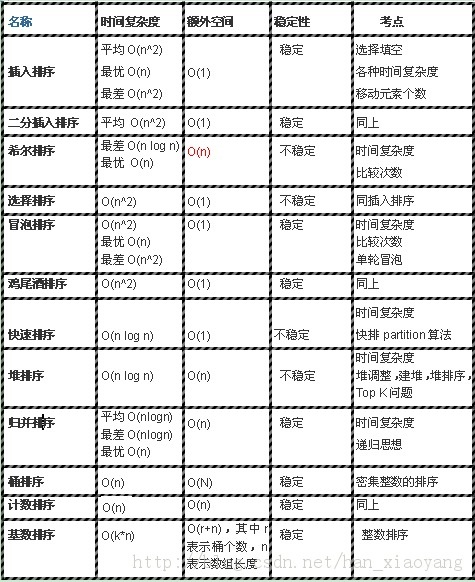9
0

Flash实战技能应用从入门到精通
ComponentOne Studio for WinForms基础课程

8楼  Mars_NAVY 2014-11-20 17:22发表 [回复]7楼  flying_2015 2014-09-11 18:43发表 [回复]6楼  shirley_sherry 2014-09-09 16:30发表 [回复]5楼  duqi_2009 2014-08-27 16:01发表 [回复]4楼  DROfanghuang 2014-08-23 15:08发表 [回复]3楼  dtrs001 2014-08-20 08:28发表 [回复]if(p1 >= arr+len)
return arr;

2楼  ikweesung 2013-11-29 14:48发表 [回复][cpp]  view plain copy
1. void quicksort(int *arr, int left, int right)
2. {
3.   int i,j,v;
4.   if(left<right)
5.   {
6.     i=left;j=right;
7.     v=arr[i]; //准备以本次最左边的元素值为标准进行划分，先保存其值
8.     do
9.     {
10.       while(arr[j]>v && i<j)
11.         j--;        //从右向左找第1个小于标准值的位置j
12.       if(i<j)                               //找到了，位置为j
13.       {
14.         arr[i] = arr[j];
15.         i++;
16.       }           //将第j个元素置于左端并重置i
17.       while(arr[i]<v && i<j)
18.         i++;      //从左向右找第1个大于标准值的位置i
19.       if(i<j)                       //找到了，位置为i
20.       {
21.         arr[j] = arr[i];
22.         j--;
23.       }           //将第i个元素置于右端并重置j
24.     }while(i!=j);
25.     arr[i] = v;         //将标准值放入它的最终位置,本次划分结束
26.     quicksort(arr, left, i-1);     //对标准值左半部递归调用本函数
27.     quicksort(arr, i+1, right);    //对标准值右半部递归调用本函数
28.   }
29. }
Re:  寒小阳 2013-11-30 09:07发表 [回复]1楼  _IdleMind 2013-10-20 10:24发表 [回复]Re:  寒小阳 2013-10-20 21:06发表 [回复]• 用 户 名：
• peach90
•
* 以上用户言论只代表其个人观点，不代表CSDN网站的观点或立场
##### 核心技术类目
• 个人资料
•
• 访问：180327次
• 积分：2428
• 等级：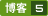• 排名：第7622名
• 原创：43篇
• 转载：1篇
• 译文：0篇
• 评论：226条
• 文章存档
• 推荐文章
• 最新评论src="http://zz.csdn.net/bin/logs.php" frameborder="0" width="0" height="0">

# 找工作知识储备(3)---从头说12种排序算法：原理、图解、动画视频演示、代码以及笔试面试题目中的应用

9366人阅读  评论(10)  收藏  举报

目录(?)[-]

作者：寒小阳

时间：2013年9月。
声明：版权所有，转载请注明出处，谢谢。

# 0、前言

从这一部分开始直接切入我们计算机互联网笔试面试中的重头戏算法了，初始的想法是找一条主线，比如数据结构或者解题思路方法，将博主见过做过整理过的算法题逐个分析一遍(博主当年自己学算法就是用这种比较笨的刷题学的，囧)，不过又想了想，算法这东西，博主自己学的过程中一直深感，基础还是非常重要的，很多难题是基础类数据结构和题目的思想综合发散而来。比如说作为最基本的排序算法就种类很多，而事实上笔试面试过程中发现掌握的程度很一般，有很多题目，包括很多算法难题，其母题或者基本思想就是基于这些经典算法的，比如说快排的partition算法，比如说归并排序中的思想，比如说桶排序中桶的思想。
这里对笔试面试最常涉及到的12种排序算法(包括插入排序、二分插入排序、希尔排序、选择排序、冒泡排序、鸡尾酒排序、快速排序、堆排序、归并排序、桶排序、计数排序和基数排序)进行了详解。每一种算法都有基本介绍、算法原理分析、图解/flash演示/视频演示、算法代码、笔试面试重点分析、笔试面试题等板块，希望能帮助大家真正理解这些排序算法，并能使用这些算法的思想解决一些题。不多说了，下面就进入正题了。

# 一、插入排序

## 1）算法简介

插入排序（Insertion Sort）的算法描述是一种简单直观的排序算法。它的工作原理是通过构建有序序列，对于未排序数据，在已排序序列中从后向前扫描，找到相应位置并插入。插入排序在实现上，通常采用in-place排序（即只需用到O(1)的额外空间的排序），因而在从后向前扫描过程中，需要反复把已排序元素逐步向后挪位，为最新元素提供插入空间。

## 2）算法描述和分析

一般来说，插入排序都采用in-place在数组上实现。具体算法描述如下：

1、从第一个元素开始，该元素可以认为已经被排序

2、取出下一个元素，在已经排序的元素序列中从后向前扫描

3、如果该元素（已排序）大于新元素，将该元素移到下一位置

4、重复步骤3，直到找到已排序的元素小于或者等于新元素的位置

5、将新元素插入到该位置后

6、重复步骤2~5

如果目标是把n个元素的序列升序排列，那么采用插入排序存在最好情况和最坏情况。最好情况就是，序列已经是升序排列了，在这种情况下，需要进行的比较操作需(n-1)次即可。最坏情况就是，序列是降序排列，那么此时需要进行的比较共有n(n-1)/2次。插入排序的赋值操作是比较操作的次数减去(n-1)次。平均来说插入排序算法复杂度为O(n^2)。因而，插入排序不适合对于数据量比较大的排序应用。但是，如果需要排序的数据量很小，例如，量级小于千，那么插入排序还是一个不错的选择。 插入排序在工业级库中也有着广泛的应用，在STL的sort算法和stdlib的qsort算法中，都将插入排序作为快速排序的补充，用于少量元素的排序（通常为8个或以下）。

## 3）算法图解、flash演示、视频演示

### 图解：### 视频：插入排序舞蹈

http://v.youku.com/v_show/id_XMjU4NTY5MzEy.html